# H(6,4) Hamming Graph

The Second DIMACS Implementation Challenge: 1992-1993

Originator: Panos Pardalos

NP Hard Problems: Maximum Clique, Graph Coloring, and Satisfiability, The Second DIMACS Implementation Challenge: 1992-1993.

A Hamming graph with parameters n and d has a node for each binary vector of length n. Two nodes are adjacent if and only if the corresponding bit vectors are hamming distance at least d apart.

## Examples

### Basic Examples

Retrieve the graph:

 In:=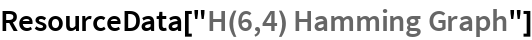Out=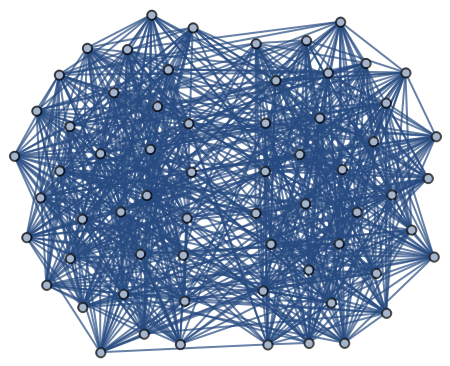Summary properties:

 In:=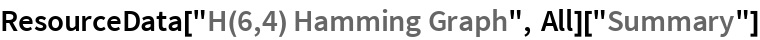Out=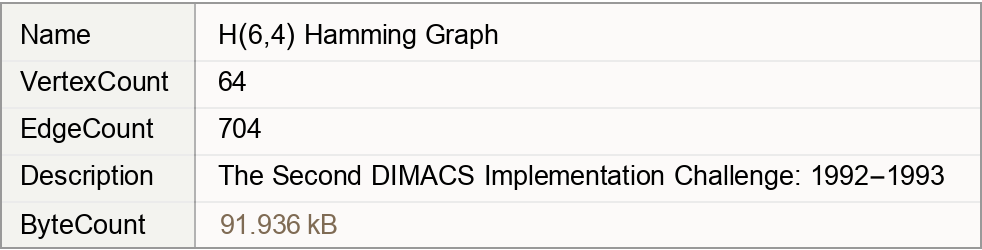### Basic Applications

Find the maximum clique:

 In:=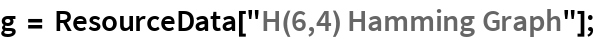In:=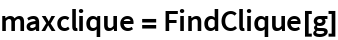Out=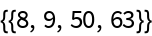Show the maximum clique:

 In:=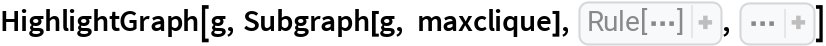Out=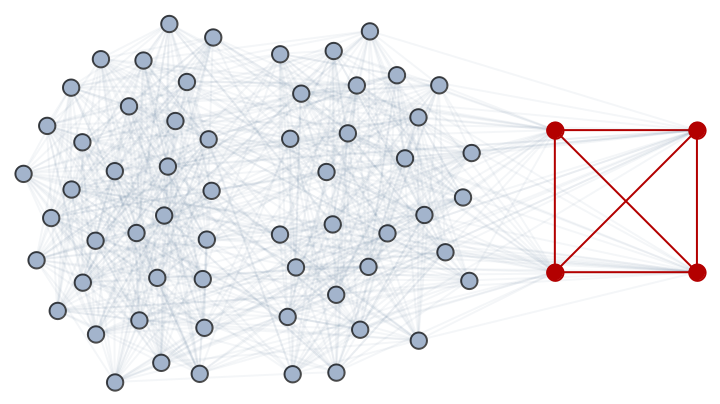Wolfram Research, "H(6,4) Hamming Graph" from the Wolfram Data Repository (2019)